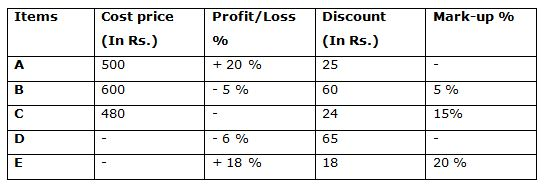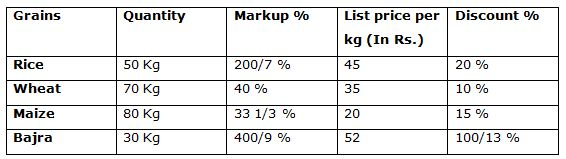# Quantitative Aptitude Questions (Data Interpretation) for SBI PO Mains 2018 Day-148

Dear Readers, SBI is conducting Online Examination for the recruitment of probationary officer. To enrich your preparation here we have providing new series of Data Interpretation – Quantitative Aptitude Questions. Candidates those who are appearing in SBI PO Mains Exams can practice these Quantitative Aptitude average questions daily and make your preparation effective.

Click “Start Quiz” to attend these Questions and view Solutions

Directions (1 – 5): Study the following information carefully and answer the given questions:

Following table shows different types of items sold by the shopkeeper and its cost price, discount, Profit/Loss % and Mark-up % also given.1. Find the ratio between the mark-up % of Item A to that of Item E?

a) 3: 5

b) 2: 3

c) 5: 4

d) 6: 7

e) None of these

2. Find the profit/loss % earned by selling the Item C?

a) 10 %

b) 14 %

c) 20 %

d) 18 %

e) None of these

3. If the cost price of Item A to that of Item D is in the ratio of 10: 13, then find the difference between the marked price of Item D to that of Item B?

a) Rs. 58

b) Rs. 64

c) Rs. 72

d) Rs. 46

e) None of these

4. The cost price of Item E is 80 % more than the cost price of Item A. The selling price of Item E is 160 less than twice the selling price of Item D. Find the cost price of Item D?

a) Rs. 700

b) Rs. 650

c) Rs. 800

d) Rs. 850

e) None of these

5. Find the average selling price of Item A, B and C?

a) Rs. 584

b) Rs. 572

c) Rs. 566

d) Rs. 528

e) None of these

Directions (6 – 10): Study the following information carefully and answer the given questions:

Following table shows the different types of grains and the quantity of different grains and markup % and list price per kg and discount % also given.6. Find the ratio between the cost price per kg of Rice to that of cost price per kg of Wheat

a) 5: 3

b) 7: 5

c) 6: 11

d) 9: 13

e) None of these

7. Find the profit of amount earned by selling the given quantity of Maize?

a) Rs. 180

b) Rs. 200

c) Rs. 225

d) 160

e) None of these

8. Find the difference between the total selling price of Maize and Bajra?

a) Rs. 90

b) Rs. 75

c) 80

d) Rs. 55

e) None of these

9. Total selling price of Rice is what percentage of total selling price of Bajra?

a) 125 %

b) 105 %

c) 115 %

d) 80 %

e) None of these

10. Find the total cost price of Wheat and Maize together?

a) Rs. 3125

b) 2950

c) Rs. 3580

d) Rs. 3750

e) None of these

Directions (Q. 1 – 5):

Selling price of Item A = 500*(120/100) = Rs. 600

Marked price of Item A = 600 + 25 = Rs. 625

CP*(100 + Mark-up %)/100 = MP

500*(100 + Mark-up %)/100 = 625

(100 + Mark-up %) = 625/5

(100 + Mark-up %) = 125

Mark-up % of Item A = 125 = 100 = 25 %

Required ratio = 25: 20 = 5: 4

Marked price of Item C

= > 480*(115/100) = Rs. 552

Selling price of Item C = 552 – 24 = Rs. 528

According to the question,

480*(x/100) = 528

x = 528*(100/480) = 110

So, Profit % = 10 %

Marked price of Item B = 600*(105/100) = Rs. 630

The ratio of cost price of Item A to that of Item D = 10: 13

10’s = 500

1’s = 50

Cost price of Item D = 13’s = Rs. 650

Selling price of Item D = 650*(94/100) = Rs. 611

Marked price of Item D = 611 + 65 = Rs. 676

Required difference = 676 – 630 = Rs. 46

The cost price of Item E = (180/100)*500 = Rs. 900

The Selling Price of Item E = 900*(118/100) = Rs. 1062

The Selling price of Item E = 2*Selling price of Item D – 160

1062 + 160 = 2* Selling price of Item D

1222/2 = Selling price of Item D

Selling price of Item D = Rs. 611

Cost price of Item D = 611*(100/94) = Rs. 650

The selling price of Item A = 500*(120/100) = Rs. 600

The selling price of Item B = 600*(95/100) = Rs. 570

The Marked price of Item C = 480*(115/100) = Rs. 552

The selling price of Item C = 552 – 24 = Rs. 528

Required average = (600 + 570 + 528)/3 = 1698/3 = Rs. 566

Directions (Q. 6 – 10):

Cost price*[(100 + Markup %)/100] = List price

The cost price per kg of Rice

CP*(100 + (200/7))/100 = 45

CP *(900/700) = 45

CP = 45*(7/9) = Rs. 35

The cost price per kg of Wheat

CP*(100 + 40)/100 = 35

CP = 35*(100/140) = 25

Required ratio = 35: 25 = 7: 5

The cost price per kg of Maize

CP*(100 + 100/3)/100 = 20

CP*(400/300) = 20

CP = 20*(3/4) = Rs. 15

The selling price per kg of Maize

LP*(85/100) = SP

20*(17/20) = SP

SP = Rs. 17

Profit per kg of Maize = 17 – 15 = Rs. 2

Total profit = 2*80 = Rs. 160

The cost price per kg of Maize

CP*(100 + 100/3)/100 = 20

CP*(400/300) = 20

CP = 20*(3/4) = Rs. 15

The selling price per kg of Maize

LP*(85/100) = SP

20*(17/20) = SP

SP = Rs. 17

Total selling price of Maize = 17*80 = Rs. 1360

The cost price per kg of Bajra

CP*(100 + 400/9)/100 = 52

CP*(1300/900) = 52

CP = 52*(9/13) = Rs. 36

The selling price per kg of Bajra

LP*(1200/1300) = SP

SP = 52*(12/13) = Rs. 48

Total selling price of Bajra = 48*30 = Rs. 1440

Required difference = 1440 – 1360 = Rs. 80

The cost price per kg of Rice

CP*(100 + (200/7))/100 = 45

CP *(900/700) = 45

CP = 45*(7/9) = Rs. 35

The selling price per kg of Rice

LP*(80/100) = SP

SP = 45*(4/5) = Rs. 36

Total selling price of Rice = 36*50 = Rs. 1800

The cost price per kg of Bajra

CP*(100 + 400/9)/100 = 52

CP*(1300/900) = 52

CP = 52*(9/13) = Rs. 36

The selling price per kg of Bajra

LP*(1200/1300) = SP

SP = 52*(12/13) = Rs. 48

Total selling price of Bajra = 48*30 = Rs. 1440

Required % = (1800/1440)*100 = 125 %

The cost price per kg of Wheat

CP*(100 + 40)/100 = 35

CP = 35*(100/140) = 25

Total cost price of Wheat = 25*70 = Rs. 1750

The cost price per kg of Maize

CP*(100 + 100/3)/100 = 20

CP*(400/300) = 20

CP = 20*(3/4) = Rs. 15

Total cost price of Maize = 15*80 = Rs. 1200

The total cost price of Wheat and Maize together

= > 1750 + 1200

= > Rs. 2950

Daily Practice Test Schedule | Good Luck

 Topic Daily Publishing Time Daily News Papers & Editorials 8.00 AM Current Affairs Quiz 9.00 AM Quantitative Aptitude “20-20” 11.00 AM Vocabulary (Based on The Hindu) 12.00 PM General Awareness “20-20” 1.00 PM English Language “20-20” 2.00 PM Reasoning Puzzles & Seating 4.00 PM Daily Current Affairs Updates 5.00 PM Data Interpretation / Application Sums (Topic Wise) 6.00 PM Reasoning Ability “20-20” 7.00 PM English Language (New Pattern Questions) 8.00 PM